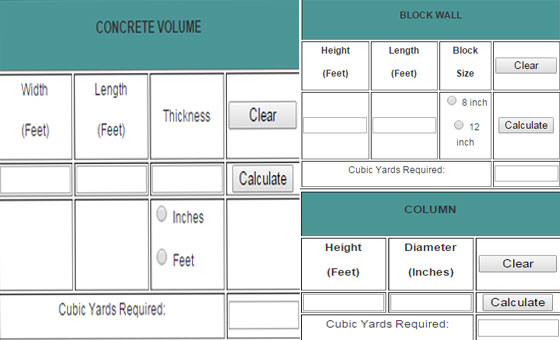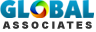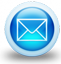# Usefulness & usage of concrete materials calculators

## Home » Plan Take off

The Concrete Materials Calculators contain the quantities of various concrete mix (cement/all-in-ballast/sharp sand/gravel etc.) essential for providing a definite volume of finished concrete.

The concrete calculators make an allowance concerning the material losses volume once it is combined to produce concrete.

The Concrete Material Calculators can be applied for making estimates in three major areas:

In order to apply the Concrete Volume Calculator, just provide the width, length, and thickness concerning pour, select the unit of measurement (feet/inches) for the thickness. After that, press on the Calculate button. The calculator will automatically compute the required number of cubic yards of concrete.

To apply the Block Wall Calculator, just put in the height and length of the wall, select the size of the wall that will be filled up (8-inch or 12-inch wall), then press the Calculate button. The calculator will automatically calculate the number of required cubic yards. Note that this is an estimate. There occurs crucial deviation in fill space regarding diverse blocks. This calculator takes into consideration the application of double open-ended bond beam blocks.

In order to utilize the Concrete Column Calculator, just indicate the height and diameter of the column, and press the calculate button. The calculator will instantly & automatically demonstrate the required number of cubic yards.Ref: concrete.com/

# Takeoff Tools